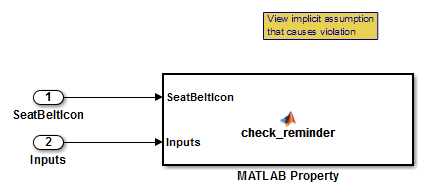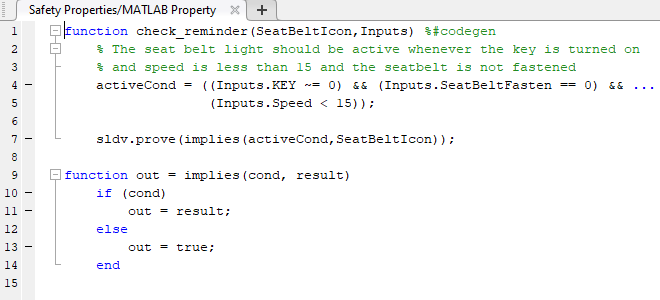# sldv.assume

Proof assumption function for Stateflow charts and MATLAB Function blocks

## Syntax

``sldv.assume(expr)``

## Description

example

````sldv.assume(expr)` specifies that `expr` is true for every evaluation while proving properties. Use any valid Boolean expression for `expr`.```

This function has no output and no impact on its parenting function, other than any indirect side effects of evaluating `expr`. If you issue this function from the MATLAB® command line, the function has no effect.

Intersperse `sldv.assume` proof assumptions within MATLAB code or separate the assumptions into a verification script.

The Proof assumptions option in the Property proving pane applies to the proof assumptions represented by the `sldv.assume` function and by the Proof Assumption block.

## Examples

collapse all

Specify a property proof objective and proof assumption in the `sldvdemo_sbr_verification` model by using a MATLAB Function block.

Open the `sldvdemo_sbr_verification` model and save it as `ex_sldvdemo_sbr_verification`.

Open the Safety Properties subsystem.Open the MATLAB Property block, which is a MATLAB Function block.At the end of the `check_reminder` function definition, add `sldv.assume(Inputs.KEY==0 | 1);` so that the last two lines of the function definition are:

```sldv.prove(implies(activeCond, SeatBeltIcon)); sldv.assume(Inputs.KEY==0 | 1);```

To save the updated code, in the Editor tab, click Save and close the editor.

To prove the safety properties, in the Simulink® Editor, select the Safety Properties subsystem. On the Design Verifier tab, click .

Alternatively, in the Simulink Editor, you can right-click the Safety Properties subsystem and select Design Verifier > Prove Subsystem Properties.

## Input Arguments

collapse all

MATLAB expression, for example, `x > 0`.

## Alternatives

Instead of using the `sldv.assume` function, you can insert a Proof Assumption block in your model. Using `sldv.assume` instead of a Proof Assumption block offers several benefits, described in What Is Property Proving?.

When proving models by using MATLAB for code generation, you can also constrain signal values without using the `sldv.assume` function. Using `sldv.assume` instead of directly using MATLAB for code generation eliminates the need to:

• Express the assumption by using a Simulink block.

• Explicitly connect the assumption output to a Simulink block.